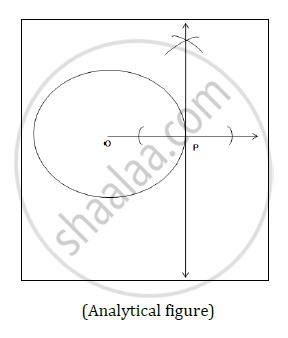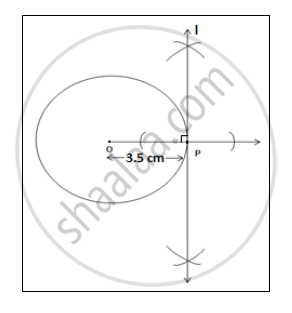# Draw a Tangent at Any Point ‘P’ on the Circle of Radius 3.5 Cm and Centre O. - Geometry

Draw a tangent at any point ‘P’ on the circle of radius 3.5 cm and centre O.

#### Solution

Construction:-Steps of construction:-

1. Draw a circle with centre O and radius 3.5cm.
2. Take any point ‘P’ on the circle and draw ray OP.
3. Draw a line perpendicular to ray OP at the point P. Name that line as ‘l’ which is tangent to the circle.Concept: Construction of Tangent to the Circle from the Point on the Circle
Is there an error in this question or solution?